# Advanced VLOOKUP formula examples: nested vlookup with multiple criteria, two-way lookup

In this second part of our Excel VLOOKUP tutorial, we will explore a few examples that will help you harness the power of VLOOKUP to cope with the most challenging Excel tasks. The samples imply that you know the basics of how this Excel function works. If not, you might want to start with Part 1 that explains VLOOKUP syntax and general usages.

Well, let's have a closer look at the following VLOOKUP formula examples:

## Excel vlookup with multiple criteria

The Excel VLOOKUP function is really great when it comes to searching across a database for a certain value. However, it lacks an important feature - its syntax allows for one lookup value only. But what if you want to look up by several conditions? The solution follows below : )

#### Example 1. Look up with 2 different criteria

Suppose you have a list of orders and you want to find the Qty. based on 2 criteria - "Customer Name" and "Product". A complicating factor is that each customer ordered multiple products, as you see in the table below: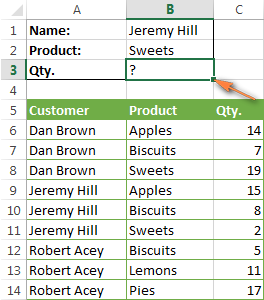A usual VLOOKUP formula won't do in this scenario, because it returns the first found value matching the lookup value you specify. So, if you want to know the quantity of "Sweets" ordered by "Jeremy Hill" and you write the formula `=VLOOKUP(B1,\$A\$5:\$C\$14,3,FALSE)`, it will bring you "15" corresponding to "Apples" since this is the first match.

An easy workaround is creating an additional column that concatenates all the criteria you want, the Customer and Product columns in this sample. Remember, the concatenated column should always be the left-most column in your lookup range because this is where Excel VLOOKUP always searches for the lookup value.

So, you add a helper column to your table and copy a formula like this `=B2&C2` across that column (or `=B2&" "&C2` if you want to separate the concatenated values with a space to make the data more readable).

And then, you can use a simple VLOOKUP formula similar to this:

`=VLOOKUP("Jeremy Hill Sweets",\$A\$5:\$C\$14,3,FALSE)`
or
`=VLOOKUP(B1,\$A\$5:\$C\$14,3,FALSE)`

Where cell B1 contains your concatenated lookup value (lookup_value) and 3 is the number of the column containing the data you want to find (col_index_num).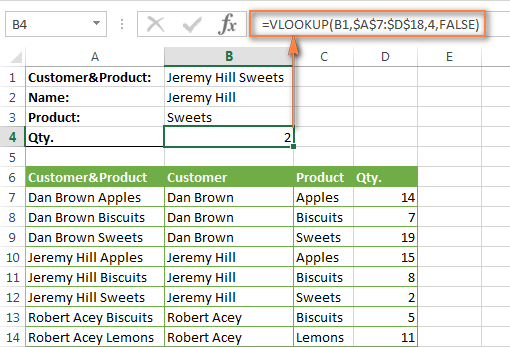#### Example 2. Vlookup with 2 criteria from a different worksheet

If you need to update your main table by pulling data from another table (different worksheet or workbook), then you can concatenate the lookup values directly in the formula that you enter in your main table.

The same as in the example above, you will need to add a helper column to your lookup table with concatenated lookup values and this should be the left-most column of the lookup range.

So, your VLOOKUP formula may look like this:

`=VLOOKUP(B2&" "&C2,Orders!\$A&\$2:\$B\$2,4,FALSE)`.

Where columns B and C contain the customer names and product names, respectively, and Orders!\$A&\$2:\$B\$2 is your lookup table in another worksheet.

Tip. To make the formula easier-to-read, you can create a named range for the lookup table, and your formula will get simplified to this: `=VLOOKUP(B2&" "&C2,Orders,4,FALSE)`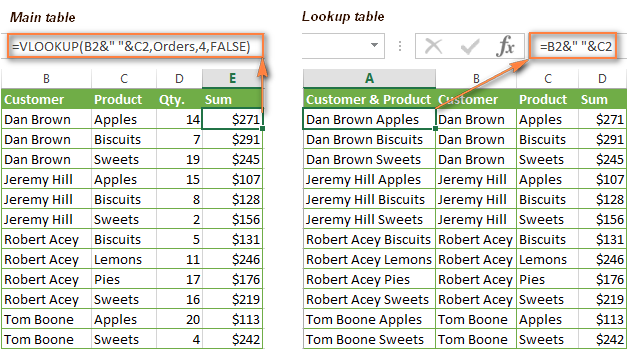Note. For the formula to work, the left-most column of your lookup table must contain the look up values concatenated exactly as in your lookup criteria. As you see in the screenshot above, we concatenated values with a space in the lookup table, so we do the same in the lookup criteria of the VLOOKUP formula (B2&" "&C2).

Also, please remember about Excel VLOOKUP's limit of 255 characters. The VLOOKUP function cannot search for a lookup value containing more than 255 chars. So, keep this number in mind and make sure the total length of your lookup criteria does not exceed this limit.

I agree that adding an additional column is not a very elegant solution and is not always acceptable. You can actually do without the "helper column", but in this case you would need a far more complex formula with a combination of INDEX and MATCH functions.

Download this sample (VLOOKUP with multiple criteria).

## How to use VLOOKUP to get 2nd, 3rd, 4th, etc. matching values

As you already know, Excel VLOOKUP can fetch only one matching value, more precisely, the first found item. But what if there are several matches in your lookup array and you want to get the 2nd or 3rd occurrence? Moreover, what if you want to pull all matching values? The task sounds quite intricate, but the solution does exist!

Suppose, you have customer names in one column and the products they purchased in another. And now, you want to find the 2nd, 3rd, or 4th product purchased by a given customer.

The simplest way is to add a helper column before the Customer Names column and populate it with the customer's name and occurrence number, e.g. "John Doe1", "John Doe2" etc. The following COUNTIF formula does the trick (assuming that the customer names are in column B):

`=B2&COUNTIF(\$B\$2:B2,B2)`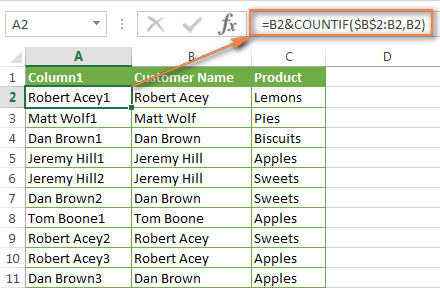After that, you can use a usual VLOOKUP formula to find the corresponding order. For example:

• Find the 2nd product purchased by Dan Brown:
`=VLOOKUP("Dan Brown2",\$A\$2:\$C\$16,3,FALSE)`
• Find the 3rd product purchased by Dan Brown:
`=VLOOKUP("Dan Brown3",\$A\$2:\$C\$16,3,FALSE)`

Naturally, you can enter a cell reference instead of text in the lookup value, as you see in the screenshot below: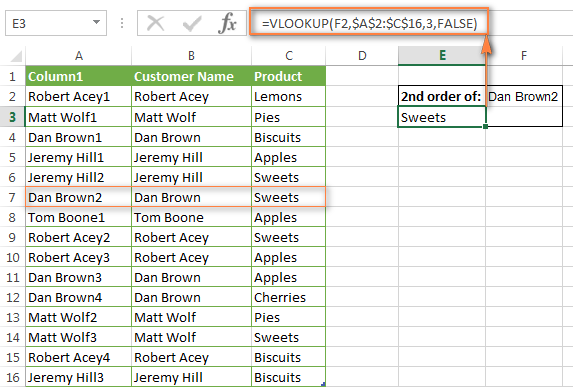If you are looking for the 2nd occurrence only, you can do without the helper column by creating a more complex VLOOKUP formula:

`=IFERROR(VLOOKUP(\$F\$2,INDIRECT("\$B\$"&(MATCH(\$F\$2,Table4[Customer Name],0)+2)&":\$C16"),2,FALSE),"")`

In the formula:

• \$F\$2 - the cell with the customer name (it is constant, please notice the absolute cell references);
• \$B\$ - the "Customer Names" column;
• Table4[Customer Name] - lookup column in the table or a lookup range;
• \$C16 - the last (bottom-left) cell of your lookup table.
Note. This formula finds the second matching value only. If you have to get other occurrences, please proceed with the previous solution.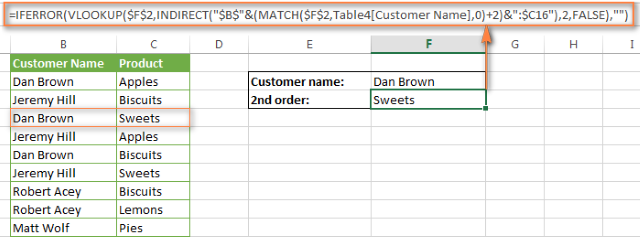Download this sample (VLOOKUP to get 2nd, 3rd, 4th, etc. matching values).

If you want to get the list of all matching values, the VLOOKUP function cannot help, because it can return only one value at a time, alas. But Excel's INDEX function can handle this scenario and I will show you the formula in the next example.

### How to get all occurrences of lookup value (duplicates)

As mentioned above, the Excel VLOOKUP function cannot get duplicate instances of the lookup value. To do this, you would need a far more complex array formula consisting of several Excel functions such as INDEX, SMALL and ROW.

For instance, the below formula finds all instances of the value in cell F2 in the lookup range B2:B16, and returns values from column C in the same rows:

`{=IFERROR(INDEX(\$C\$2:\$C\$16, SMALL(IF(\$F\$2=B2:B16, ROW(C2:C16)-1,""), ROW()-3)),"")}`

Copy the below formula to several adjacent cells, e.g. cells F4:F8 as demonstrated in the screenshot below. The number of cells where you copy the formula should be equal to or larger than the maximum number of possible duplicate entries. Also, remember to press Ctrl + Shift + Enter to enter an array formula correctly.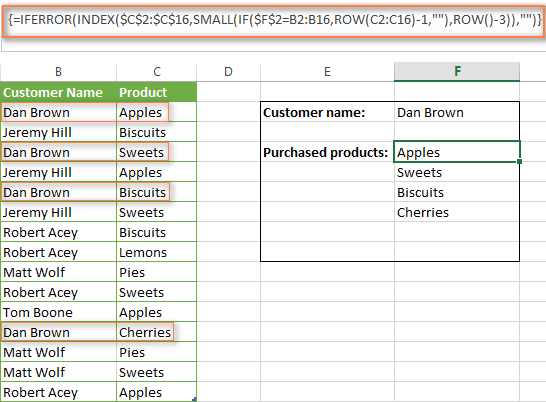If you are curious to know the underlying logic, let's drill down into the formula a bit:

Part 1. `IF(\$F\$2=B2:B16,ROW(C2:C16)-1,"")`

`\$F\$2=B2:B16` - compares the value in cell F2 with each value in the range B2:B16. If a match is found, `ROW(C2:C16)-1` returns the number of the corresponding row (-1 is used to deduct the header row). If the compared values do not match, the IF function returns an empty string.

The result of the IF function is the following array {1, "", 3, "", 5, "", "", "", "", "", "",12, "", "", ""}

Part 2. `ROW()-3`

In this case, the ROW function acts as an incremental counter. Since the formula is copied into cells F4:F9, we add -3 for the function to return 1 for cell F4 (row 4 minus 3), 2 for cell F5 (row 5 minus 3), etc.

Part 3. `SMALL(IF(\$F\$2=\$B\$2:\$B\$16,ROW(\$C\$2:\$C\$16)-1,""),ROW()-3))`

The SMALL function returns the k-th smallest value in a data set. In our case, the position (from the smallest) to return is defined by the ROW function (Part 2). So, for cell F4, the function SMALL({array},1) returns the 1st (smallest) element of the array, i.e. 1. For cell F5, it returns the 2nd smallest element of the array, which is 3, and so on.

Part 4. `INDEX(\$C\$2:\$C\$16, SMALL(IF(\$F\$2=\$B\$2:\$B\$16, ROW(\$C\$2:\$C\$16)-1,""), ROW()-3))`

The INDEX function simply returns the value of a specified cell in the array C2:C16. For cell F4, `INDEX(\$C\$2:\$C\$16,1)` returns "Apples"; for cell F5, `INDEX(\$C\$2:\$C\$16,3)` returns "Sweets", etc.

Part 5. `IFERROR()`

Finally, we wrap the formula in the IFERROR function, because you would hardly want to see N/A error messages in your worksheet when the number of cells where you've copied the formula is greater than the number of duplicate occurrences of the lookup value.

Download this sample (Get all duplicate values in the lookup range).

## How to do two-way lookup in Excel

2-dimensional lookup in Excel (aka matrix lookup or two-way lookup) is looking up across both rows and columns. In other words, you search for a value at the intersection of a certain row and column.

So, let's use our 'Monthly Sales' table again and write a VLOOKUP formula that finds how many lemons were sold in March.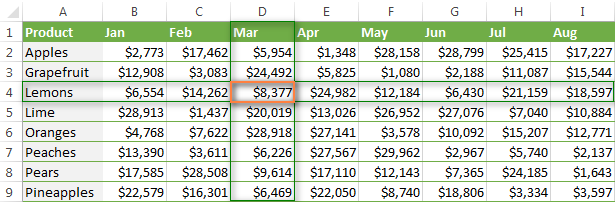You can do two-way lookup in a few different ways. So, look through the possible alternatives below and choose your winner : )

#### VLOOKUP & MATCH functions

You can use a liaison of the VLOOKUP and MATCH functions to cross-reference two fields in a database, Product (row) and Month (column) in this example:

`=VLOOKUP("Lemons",\$A\$2:\$I\$9,MATCH("Mar",\$A\$1:\$I\$1,0),FALSE)`

The formula above is a usual Excel VLOOKUP function that searches for the exact match of "Lemons" in cells A2 through I9. But, since you do not know in which exactly column March's sales are, you cannot supply the column number in the third argument of your VLOOKUP formula. Instead, you use the MATCH function to find that column.

Translated into plain English, the function `MATCH("Mar",\$A\$1:\$I\$1,0)` means:

• Look up "Mar" (lookup_value argument).
• Search in cells A1 to I1 (lookup_array argument).
• Return the exact match (match_type argument). By using "0" in the 3rd parameter, you tell the MATCH function to find the first value that is exactly equal to the lookup value. You can regard it as an equivalent of the False parameter in VLOOKUP.

This is how you create a lookup formula with two criteria in Excel, which is also known as a two-way, or two-dimensional lookup.

#### SUMPRODUCT function

The SUMPRODUCT function multiplies the components in the given arrays, and returns their sum:

`=SUMPRODUCT((\$A\$2:\$A\$9="Lemons")*(\$A\$1:\$I\$1="Mar"),\$A\$2:\$I\$9)`

#### INDEX & MATCH functions

In will explain these functions in full detail in the next article, so you can simply grab the formula for now :

`=INDEX(\$A\$2:\$I\$9, MATCH("Lemons",\$A\$2:\$A\$9,0), MATCH("Mar",\$A\$1:\$I\$1,0))`

#### Named Ranges & SPACE (intersection operator)

If you are not particularly fond of all those complex Excel formulas, you may like this visual and easy-to-remember way:

1. Select you table, switch to the Formulas tab and click Create from Selection.
2. Select the "Top row" and "Left column options. Microsoft Excel will create names from the values in the top row and left-most column of your selection, and you will be able to look up those names directly instead of making up a formula.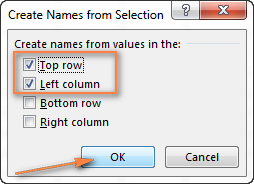3. In any empty cell, type =row_value column_value, e.g. =Lemons Mar, or vice versa =Mar Lemons.

Please remember to separate your row value and column value with a space, which acts as the intersection operator in this case.

As you type, Microsoft Excel will display a list of matching names, exactly as it does when you start typing a formula.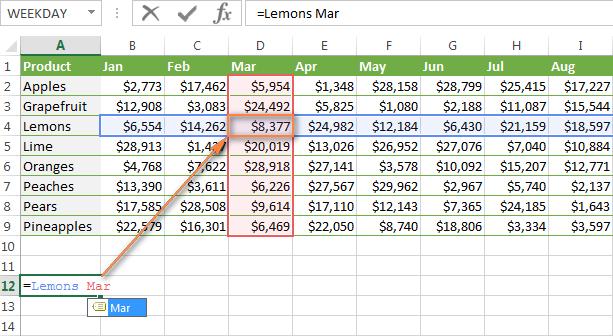4. Press the Enter key and verify the result.

All in all, whichever method you choose, the result of your two-dimensional lookup will be the same: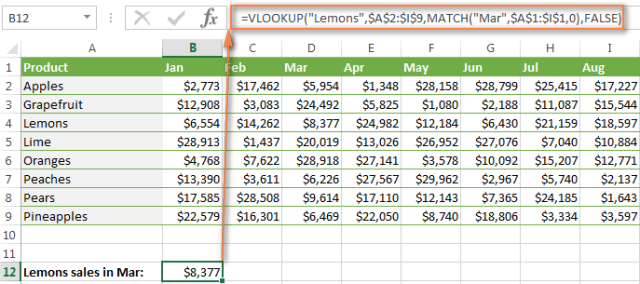Download this sample (2-way VLOOKUP).

## How to do multiple vlookups in one formula (nested vlookup)

Sometimes it may happen that your main table and lookup table do not have a single column in common, which prevents you from doing a normal vlookup. However, there exists another table, which does not contain the information you are looking for, but has one common column with your main table and another common column with your lookup table.

Let's consider the following example. You have the main table with a single column, New SKU, and you need to pull the corresponding prices from another table. Also, you have 2 lookup tables - the first contains the same New SKU numbers and product names, while the other lists the product names, prices, but the Old SKU numbers.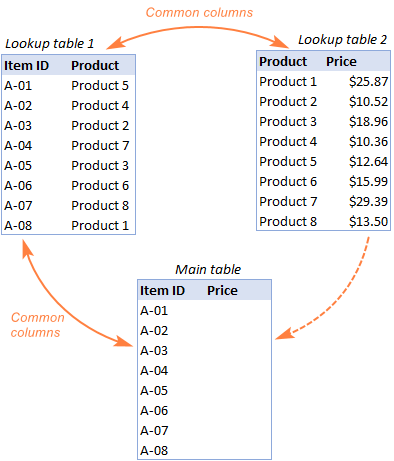To pull the prices from Lookup table 2 to the Main table, you have to perform what is known as Excel's double vlookup, or nested vlookup.

1. Create a VLOOKUP formula that finds the product name in "Lookup table 1", using a new SKU as the lookup value:

`=VLOOKUP(A2,New_SKU,2,FALSE)`

Where 'New_SKU' is a named range for \$A:\$B in "Lookup table 1", and "Lookup table 2", is column B containing the products names (please see the image above).

2. Write the formula to pull the prices from "Lookup table 2", based on the product's name, by nesting the above vlookup function in the lookup criteria:

`=VLOOKUP(VLOOKUP(A2,New_SKU,2,FALSE),Price,3,FALSE)`

Where 'Price' is a named range \$A:\$C in Lookup table 2, and 3 is column C containing the prices.

The screenshot below shows the result returned by our nested vlookup formula: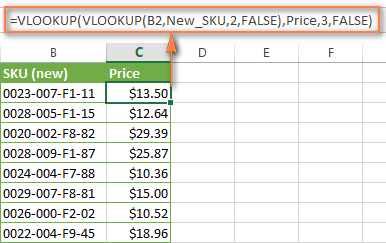Download this sample (nested VLOOKUP).

## Use VLOOKUP and INDIRECT to dynamically pull data from different sheets

To begin with, let's define what exactly "dynamically pull data from different sheets" means to make sure we are on the same page : )

In some cases, you may have data in the same format split over multiple spreadsheets and each time you want to pull matching data from a specific sheet depending on what value is entered in a given cell. I think this will be easier to understand from an example.

Suppose, you have a few regional sales reports for the same products in the same format, and you want to find the sales number for a certain region: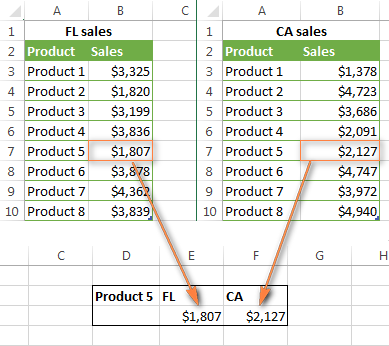If you have just a couple of regional reports, you can use a fairly simple VLOOKUP formula with an IF function to select the correct sheet for vlookup:

`=VLOOKUP(\$D\$2,IF(\$D3="FL",FL_Sales,CA_Sales),2,FALSE)`

Where:

• \$D\$2 is a cell containing the "Product Name". Note that we use absolute cell references in this case to prevent the lookup value from changing when copying the formula to other cells.
• \$D3 is a cell with the state name (use an absolute column reference and relative row if you plan to copy the formula to other cells in the same column).
• FL_Sales and CA_Sales are the table names, or named ranges, for corresponding sales reports. You can also use worksheets names and usual cell ranges, e.g. 'FL Sheet'!\$A\$3:\$B\$10, but using named ranges is much more convenient.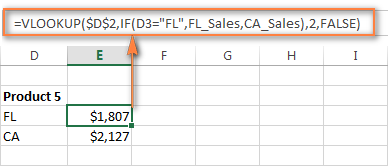However, if you have multiple lookup tables, the IF function is not the ideal solution. Instead, you can use the INDIRECT function to return the required lookup range.

As you probably know, the Excel INDIRECT function is used to indirectly reference a cell, and this is exactly what we need now. So, go ahead and replace the IF statement with the INDIRECT reference in the above formula. The following combination of VLOOKUP and INDIRECT works a treat in our case:

`=VLOOKUP(\$D\$2,INDIRECT(\$D3&"_Sales"),2,FALSE)`

Where:

• \$D\$2 is the cell containing the product name, it always remains constant due to the absolute row and column references.
• \$D3 is the cell containing the first state name, FL in our case.
• "_Sales" is the common part of your range names, or table names. Concatenated with the value in cell D3 it makes the full name of the required range. Below I'll provide some more details for those who do not have much experience with Excel's INDIRECT function.

#### INDIRECT & VLOOKUP - how it works

First off, let me remind you the syntax of the INDIRECT function: INDIRECT(ref_text, [a1])

The first parameter can be a cell reference of the A1-style or R1C1-style, a range name, or a text string. The second parameter specifies what type of reference is contained in ref_text - A1-style (TRUE or omitted) or R1C1-style (FALSE). It is A1 in our case, so we can omit the second parameter and focus solely on the first one.

Now, let's get back to our sales reports. As you remember, each report is a separate table residing in a separate sheet. For the formula to work, you need to name your tables or ranges, and all of the names should have some common part. For example, my sales reports are named: CA_Sales, FL_Sales, TX_Sales etc. As you see, there is always the _Sales part.

So, our INDIRECT function INDIRECT(\$D3&"_Sales") concatenates the value in column D and the word _Sales (with an underscore), and tells the VLOOKUP function in which exactly table to look up. That is, if you have FL in cell D3, the formula will search in the FL_Sales table, if CA - in CA_Sales table, and so on.

The result produced by your VLOOKUP and INDIRECT functions will look similar to this: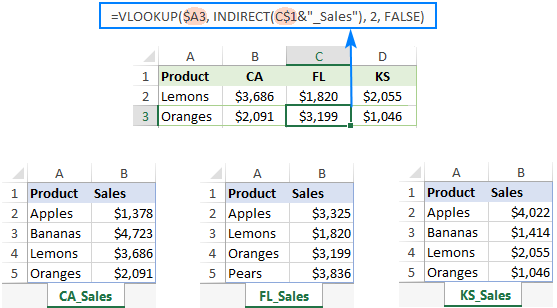If your data reside in different workbooks, you will have to add a workbook name before the named region (WorkbookName!NamedRange), for example:

`=VLOOKUP(\$D\$2,INDIRECT(\$D3&"Workbook1!_Sales"),2,FALSE)`

Note. If the INDIRECT function refers to another workbook, that workbook must be open. If the source workbook is not open, your INDIRECT formula will return the #REF! error.

Download this sample (VLOOKUP and INDIRECT).

## Merge Tables Wizard - a visual way to do vlookup in Excel

If you are not an avid fan of complex Excel formulas and you'd rather save our energies for occupations more interesting than making your way through the arcane twists of lookup criteria and arrays, you may find this merging tool really helpful.

Instead of writing formulas, the Merge Tables Wizard will ask you to supply your main table and lookup table, specify a common column or columns, and point out what info you want to update or add to the end of the main table.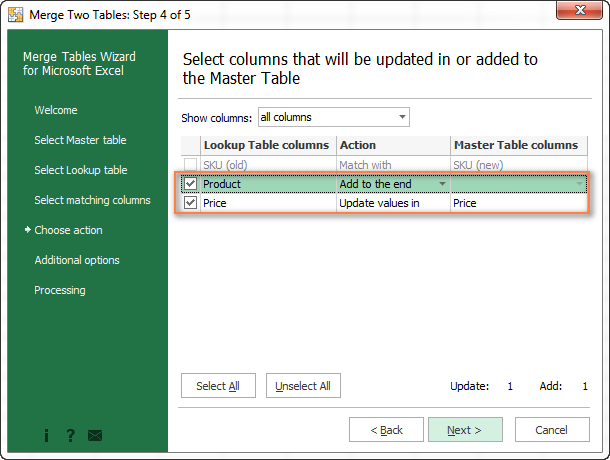Then you click Next and allow the Merge Tables Wizard a few seconds to look up, match and deliver the result. If you think you may like this add-in, you are most welcome to download a trial version : )

### 282 Responses to "Advanced VLOOKUP formula examples: nested vlookup with multiple criteria, two-way lookup"

1.Priya says:

HI

I have an excel data like following, i want to retrieve style DEC and PQR becasue all their SKU's had sale. do not want to retrieve data for styles whose all SKU's did not have sale.

How to go about it?

Style SKU Sale
ABC 111
ABC 222 Yes
ABC 333 Yes
ABC 444 Yes
ABC 555 Yes
DEF 1111 Yes
DEF 2222 Yes
DEF 3333 Yes
DEF 4444 Yes
DEF 5555 Yes
PQR 121 Yes
PQR 212 Yes
PQR 312 Yes
PQR 412 Yes
PQR 512 Yes
PQR 612 Yes
PQR 712 Yes
LMN 322 Yes
LMN 422 Yes
LMN 522 Yes
LMN 622
LMN 722

2.Sean says:

How do we check using vlookup which customer ordered Apple

Column A = Customer
Row 1A= John
Row 2A= Susan
Row 3A= Sherry

Column B = Product
Row 1B= Grapes,Apple
Row 2B= Apples,Lime
Row 3B= Pear,Pineapple

3.Ronald says:

Hi Ablebits!
Thanks Very much! Your tutorials do me great job!
My vlookup dosent work out, it returns N/A i have tried all trouble shootslike advised.
Question; Can the version of excel be an issue, besides does the fomat of the cell be a matter.
waiting
Ronald

•Svetlana Cheusheva says:

Hi Ronald,

Vlookup works in all versions of Excel, but the format of the cell can be an issue, for example a number formatted as text. You can find a list of the most common reasons for #N/A and other errors in this tutorial:
Excel VLOOKUP not working

4.KARTIK says:

how to concatenate a coloumn data from A1:A10 without using formula =CONCATENATE(A1&A2&A3&A4&A5&A6&A7&A8&A9&A10)

IS THERE ANY OTHER FORMULA FOR GOT CONCATENATE OF A1TOA10 CELLS VALUE AT ONCE

•mohammadashooryan says:

you can use cocat function in excel2019

5.Khan says:

=VLOOKUP(B\$1,RT,2,)
Kindly Explain

6.Pavan says:

Vlookup to get 2nd occurrence using Indirect function is not working. It's giving the first occurrence only. It's working in the sample file attached. But it's not working in real time scenarios.

7.SUDIP says:

Can Someone please advise me, how can i have value of one label appearing multiple times in Col-A and its return value in Col-B

For Ex

Col-A Col-B
ABC 1
ABC 2
ABC 3
XYZ 4
CBA 5
ABC 6
ABC 7
FFF 8

to other sheet like below

ABC 1
ABC 2
ABC 3
ABC 6
ABC 7

Thanks
Sudip

8.Ali says:

need a formula in vlookup for 2 scenario

9.Pradeep says:

HiHi,
I have query regarding find/search and vlookup.
I want find specific text from cell (string) and retrieve data from vlookup table by using this specific text.
e.g. “This is a boy” or “This is a girl” is in one cell.(there will more than 100 sentences) In Vlookup table Boy = Male and girl = Female.
I want display Male or Female in another cell of same sheet depends on cell string contains boy or girl.
Regards,
Pradeep

10.Shailendra Tripathi says:

=IF(ISNA(MATCH(A2,Individual!\$A\$2:\$A\$108385,0)),VLOOKUP(A2,Individual!\$A\$2:\$A\$108385,C2&"/"&C3,FALSE)," ")

Row ID Relationship Name
132361 Father Buchi Ramulu
132361 Mother Sujatha
132364 Father Mahesh
132387 Father B.Ramulu
132387 Mother Kondamma
132390 Father Anjaneyulu
132390 Mother Laxmamma

This Row ID should match with other sheet Row ID and return father & mother name "Father/Mother" in single cell.

11.Brian Higgins says:

Hi,
In the below formula what is the ! symbol for?
=LOOKUP(D26,'B:\HPServices\Current\[Material Price Book.xlsx]Material Pricing'!\$B2:\$B196,'B:\HPServices\Current\[Material Price Book.xlsx]Material Pricing'!\$H2:\$H196)

Brian

12.COLIN ROBERTS says:

hi
i am learning excel and i am using a football table,what i`m stuck on is when i enter the weeks results i now want to be able to look for a teams name and be able to bring up its last 5 results to show its current form,i can do this with a pivot table but its time consuming doing every team i was wondering if it can be done to show the whole leagues current form

thanks
colin

13.Paul says:

My data is given below:

A B C D
Product Rohim korim jodu
TV 100 200 300
AC 301 302 303
FREEZER 3002 3005 3009

After enter the following data I need result (?)
A B C
Rohim TV ??? (result should be 100)
Korim AC ??? (result should be 302)

How can I do it with vlookup or other formula in column C . Pls help.

14.AMIT MANDAL says:

Hi,

How can i get different variable with same answer
For example

Name Roll nol
amit 1
vikas 2
ajeet 3
raj 4
manish 5
sanjeet 6
suraj 7
amit 8

15.Pranner says:

Wonderful tutorials! How helpful!
I've looked for a vlookup formula to add figures with common letter code down columns. Example (Letters col. A, \$Values col. B):

AAA \$330
AAA \$600
AAA \$270
BBB \$300
CCC \$100
CCC \$120

On my separate spreadsheet, I would like to add all AAA values in one cell, \$1,200; all BBB \$300; CCC \$220, etc.

Thanks!

16.JAY says:

Hi,
i am having some product in my master sheet. and i am having month wise sheet like sheet 1= jan,sheet2= fab,...now i enter randomly product name in month wise sheet and his corresponding value. for using vlookup formula i collect corresponding product value from sheet1= jan by(=VLOOKUP(A9,'jan'!B7:F26,5,0). so now i want to collect value for same product in other remaning month and adding value form all month in master sheet. please help

17.ISHANI says:

Hi madam,
i refer above all step for my case, but still not found a solution. i have 2 kind of excel sheet. when i select one date in 1st sheet need to get 2 detail from another sheet according to selection data. i try to lot of time & through the every step. but couldn'd. please help me....

18.Cheryl says:

Hi, I'm trying to create a v-lookup formula that will look at the corresponding ID in column 1 of sheet1 (which contains my formula), then lookup the ID on sheet2 and bring in the data from column 7 of sheet2 IF... column 6 = 2019. I do v-lookups between sheets all the time and have been trying to put it together with an IF formula but I haven't been able to get it to work. Can you help?

19.Neamat Ahmed says:

hey; i need your help; i have a sheet containing 500 types of equipment each equipment has 2 or three prices and i need to get from it the min price , average and max prices and returning the supplier name for each value

Ultimate Suite 2018.5 for Excel